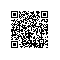# Scala入门到精通——第十九节 隐式转换与隐式参数（二）

1. 隐式参数中的隐式转换
2. 函数中隐式参数使用概要
3. 隐式转换问题梳理

## 1. 隐式参数中的隐式转换

object ImplicitParameter extends App {
//下面的代码不能编译通过
//这里面泛型T没有具体指定，它不能直接使用
//<符号进行比较
def compare[T](first:T,second:T)={
if (first < second)
first
else
second
}
}

object ImplicitParameter extends App {
//指定T的上界为Ordered[T]，所有混入了特质Ordered
//的类都可以直接的使用<比较符号进行比较
def compare[T<:Ordered[T]](first:T,second:T)={
if (first < second)
first
else
second
}
}

object ImplicitParameter extends App {
//下面代码中的(implicit order:T=>Ordered[T])
//给函数compare指定了一个隐式参数
//该隐式参数是一个隐式转换
def compare[T](first:T,second:T)(implicit order:T=>Ordered[T])={
if (first > second)
first
else
second
}
println(compare("A","B"))
}

## 2. 函数中隐式参数使用概要

//implicit关键字在下面的函数中只能出现一次
//它作用于两个参数x,y，也即x,y都是隐式参数
def sum(implicit x: Int, y: Int) = x + y
//下面的函数不合法，函数如果没有柯里化，不能期望
//implicit关键字会作用于其中一个参数
//def sum(x: Int, implicit y: Int) = x + y
//def sum(implicit x: Int, implicit y: Int) = x + y

def sum(implicit x: Int, y: Int) = x + y
//只能指定一个隐式值
//例如下面下定义的x会自动对应maxFunc中的
//参数x,y即x=3,y=3，从而得到的结果是6
implicit val x:Int=3
//不能定义两个隐式值
//implicit val y:Int=4
println(sum)

def sum(x: Int)(implicit y:Int)=x+y

def sum(x: Int)(implicit y:Int)(d:Int)=x+y+d
def sum(x: Int)(implicit y:Int)(implicit d:Int)=x+y+d

 val sum2=(implicit x:Int)=>x+1


def sum(x: Int)(implicit y:Int)=x+y
//不能定义sum的偏函数，因为它带有隐式参数
//could not find implicit value for
//parameter y: Int
//not enough arguments for method sum:
// (implicit y: Int)Int. Unspecified value parameter y.
def sum2=sum _

## 3. 隐式转换问题梳理

1 多次隐式转换问题

class RichFile(val file:File){
}

class RichFileAnother(val file:RichFile){
}

//隐式转换不会多次进行，下面的语句会报错
//不能期望会发生File到RichFile，然后RifchFile到
//RichFileAnthoer的转换
println(f)

class ClassA {
override def toString() = "This is Class A"
}
class ClassB {
override def toString() = "This is Class B"
}
class ClassC {
override def toString() = "This is  ClassC"
def printC(c: ClassC) = println(c)
}
class ClassD

object ImplicitWhole extends App {
implicit def B2C(b: ClassB) = {
println("B2C")
new ClassC
}
implicit def D2C(d: ClassD) = {
println("D2C")
new ClassC
}
//下面的代码会进行两次隐式转换
//因为ClassD中并没有printC方法
//因为它会隐式转换为ClassC（这是第一次,D2C）
//然后调用printC方法
//但是printC方法只接受ClassC类型的参数
//然而传入的参数类型是ClassB
//类型不匹配，从而又发生了一次隐式转地换(这是第二次,B2C）
//从而最终实现了方法的调用
new ClassD().printC(new ClassB)
}

object Main extends App {
class PrintOps() {
def print(implicit i: Int) = println(i);
}

implicit def str2PrintOps(s: String) = {
println("str2PrintOps")
new PrintOps
}

implicit def str2int(implicit s: String): Int = {
println("str2int")
Integer.parseInt(s)
}

implicit def getString = {
println("getString")
"123"
}
//下面的代码会发生三次隐式转换
//首先编译器发现String类型是没有print方法的
//尝试隐式转换，利用str2PrintOps方法将String
//转换成PrintOps（第一次）
//然后调用print方法，但print方法接受整型的隐式参数
//此时编译器会搜索隐式值，但程序里面没有给定，此时
//编译器会尝试调用 str2int方法进行隐式转换，但该方法
//又接受一个implicit String类型参数，编译器又会尝试
//查找一个对应的隐式值，此时又没有，因此编译器会尝试调用
//getString方法对应的字符串（这是第二次隐式转换，
//获取一个字符串，从无到有的过程）
//得到该字符串后，再调用str2int方法将String类型字符串
//转换成Int类型（这是第三次隐式转换）
"a".print
}

2 要不要用隐式转换的问题

1 即使你能轻松驾驭scala语言中的隐式转换，能不用隐式转换就尽量不用
2 如果一定要用，在涉及多次隐式转换时，必须要说服自己这样做的合理性
3 如果只是炫耀自己的scala语言能力，请大胆使用使用钉钉扫一扫加入圈子
+ 订阅